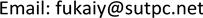1深圳市城市交通规划设计研究中心有限公司，广东 深圳

2广东省交通信息工程技术研究中心，广东 深圳1. 引言

2. 智能驾驶模型

v ˙ I D M ( s , v , Δ v ) = a [ 1 − ( v v 0 ) 4 − ( s * ( v , Δ v ) s ) 2 ] (1)

s * ( v f , Δ v ) = s 0 + v f T − v f Δ v 2 a b (2)

3. 交叉熵算法

X * = arg min P I ( X ) , X ∈ Ω (3)

l ^ ( z ) = 1 N ∑ i = 1 N I ( P I ( X i ) ≤ z ) f ( X i ) g ( X i ) (4)

X i 表示服从概率分布函数 f ( X ) 的样本， i = 1 , 2 , ⋯ , N 。 I ( P I ( X i ) ≤ z ) 表示指示变量，当事件 P I ( X i ) ≤ z 成立时取值1，其他情况取值0。当z越来越接近最优值 z * 时，满足事件 P I ( X i ) ≤ z 的样本非常少，概率 l ( z ) 的取值非常小，因此优化问题的求解转换成小概率事件的概率估计问题。

1) 迭代计数器k初始化为0，并令概率分布函数的参数 γ = γ 0 。

2) 当 k = 0 , 1 , 2 , ⋯ , 生成一系列服从概率分布函数 f ( X | γ k ) 的随机样本 { X i , k } ，( i = 1 , 2 , ⋯ , N )，并且计算各个样本的目标函数值 P I ( X i , k ) 。将得到的PI值从小到大排序，并且挑选取得最小目标值的 100 ρ % (一般 ρ 取0.05)作为重要样本，用 s b e s t 表示，同时令 z k 等于排序好的 100 ρ % 分为的目标函数值。

3) 利用重要样本 { X j , k } ( j ∈ s b e s t ) 的相关信息更新概率分布函数的参数 γ ，使得 ∑ i ln ( f ( X j , k | γ k ) ) 取最小值，用 γ k n e w 表示。更新参数的原则如下所述：

γ k + 1 = β γ k n e w + ( 1 − β ) γ k (5)

4) 计数器k加1，并返回第二步，直到满足预设的迭代停止条件。

4. 跟驰模型标定的目标函数

P I ( X ) = ∑ t = 1 T t ( ln s t c a l i ( X ) − ln s t o b s e ) 2 (6)

5. 案例分析5.1. 标定合成路径数据

5.2. 标定NGSIM路径数据

NGSIM路径数据收集的是加利福利亚州内名为Interstate 80高速公路的一段路径，时间是从下午4点到4点15分，日期是2005年4月13号。该路段由6条车道和一条汇流闸道组成。在这个例子中，我们随机挑选了该路径上的一对跟车对作为标定数据源，验证交叉熵算法在标定实测路径数据的有效性。得到的结果如表2和图2所示。

The calibration result with synthetic dat

The calibration result with NGSIM dat

6. 结束语